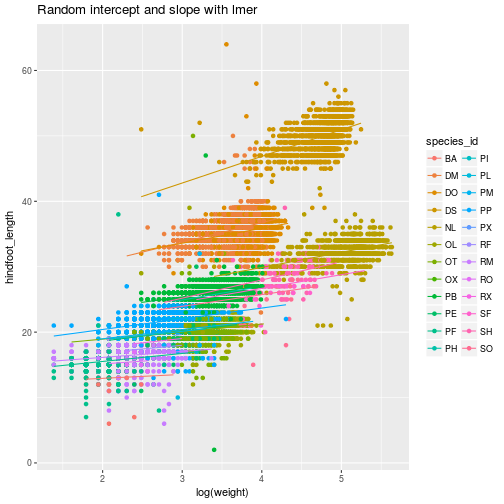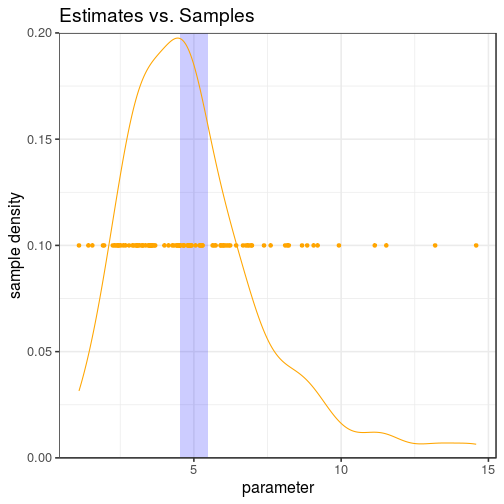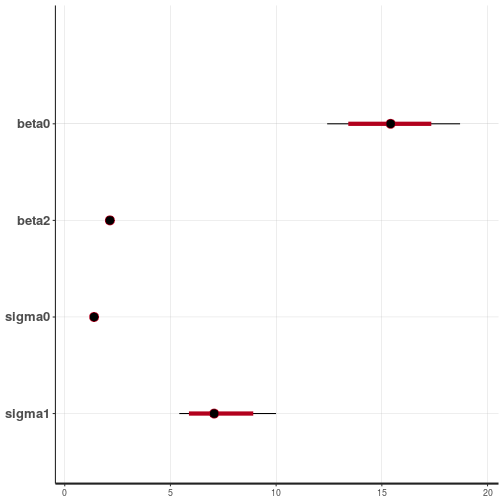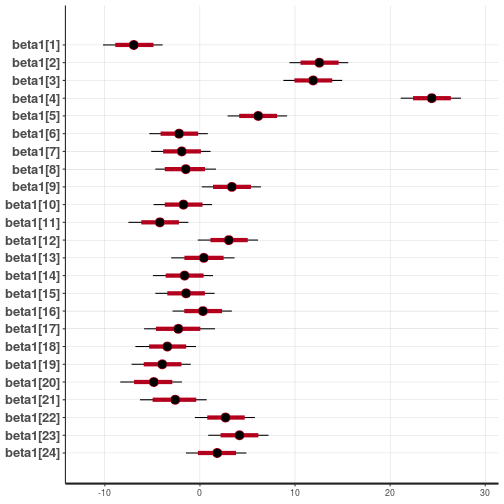# Regression

Lesson 6 with Ian Carroll

## Lesson Objectives

• Learn about R functions and extensions for statistical modeling
• Understand the “formula” part of model specification
• Introduce increasingly complex “linear models”
• Meet Stan, the MCMC sampler

## Lesson Non-objectives

(Pay no attention to these very important topics.)

• Experimental/sampling design
• Model validation
• Hypothesis tests
• Model comparison

## Specific Achievements

• Write a lm formula with an interaction term
• Use a non-gaussian family in the glm function
• Add a “random effect” to a lmer formula
• Sample parameter values for a GLM with rstan

Top of Section

## Formula Notation

The basic function for fitting a regression in R is the lm() function, standing for linear model.

> wt_len <- weight ~ hindfoot_length
> wt_len.fit <- lm(formula = wt_len,
+                  data = animals)


The lm() function takes a formula argument and a data argument, and computes the best fitting linear model (i.e. determine the optimum coefficients via least-squared-error).

### Formulas + Data

The implementation of lm() is a specific solution to a general problem: how can a human easily write down a statistical model that a machine can interpret, relate to data, and optimize through a given fitting procedure?

The lm() function requires both careful human input and correct metadata:

1. Use a unquoted “formula” expression that mixes variable names and algebra-like operators, to define a design matrix.
2. Check the variables used in the formula to complete the design matrix.
3. Compute the linear least-squares estimator and more.

Note that “the details” are left to the lm() function where possible. For example, whether a variable is a factor or numeric is read from the data frame; the formula does not distinguish between data types.

## Formula Mini-language(s)

• “base R” function lm()
• “base R” function glm()
• lme4 function lmer()
• lme4 function glmer()
• rstan models for Stan

Across different packages in R, the formula “mini-language” has evolved to allow complex model specification and fitting. Each package adds syntax to the formula or new arguments to the fitting function. On a far-distant branch of this evolution is the Stan modeling language, which provides the greatest flexibility but demands in return the most descriptive language.

Top of Section

## Linear Models

The formula requires a response variable left of a ~ and any number of predictors to its right.

 Formula Description y ~ a constant and one predictor y ~ -1 + a one predictor with no constant y ~ a + b constant and two predictors
 y ~ a:b constant and one predictor, the interaction of a factor and another variable y ~ a*b constant and three predictors y ~ a*b - a constant and two predictors y ~ (a + b + ... )^n constant and all combinations of predictors up to order n

In addition, certain functions are allowed within the formula definition.

animals <- read.csv('data/animals.csv',
na.strings = '')
fit <- lm(
hindfoot_length ~ log(weight),
data = animals)

> summary(fit)


Call:
lm(formula = hindfoot_length ~ log(weight), data = animals)

Residuals:
Min      1Q  Median      3Q     Max
-26.573  -2.976   0.987   3.483  33.738

Coefficients:
Estimate Std. Error t value Pr(>|t|)
(Intercept) -8.69213    0.14048  -61.88   <2e-16 ***
log(weight) 10.95644    0.03973  275.80   <2e-16 ***
---
Signif. codes:  0 '***' 0.001 '**' 0.01 '*' 0.05 '.' 0.1 ' ' 1

Residual standard error: 5.119 on 30736 degrees of freedom
(4811 observations deleted due to missingness)
Multiple R-squared:  0.7122,	Adjusted R-squared:  0.7122
F-statistic: 7.607e+04 on 1 and 30736 DF,  p-value: < 2.2e-16


For the predictors in a linear model, there is a big difference between factors and numbers.

fit <- lm(
log(weight) ~ species_id,
data = animals)

> summary(fit)


Call:
lm(formula = log(weight) ~ species_id, data = animals)

Residuals:
Min       1Q   Median       3Q      Max
-2.28157 -0.10063  0.02803  0.12574  1.48272

Coefficients:
Estimate Std. Error t value Pr(>|t|)
(Intercept)   2.12857    0.03110  68.448  < 2e-16 ***
species_idDM  1.62159    0.03117  52.031  < 2e-16 ***
species_idDO  1.74519    0.03134  55.690  < 2e-16 ***
species_idDS  2.63791    0.03139  84.024  < 2e-16 ***
species_idNL  2.89645    0.03170  91.373  < 2e-16 ***
species_idOL  1.29724    0.03181  40.780  < 2e-16 ***
species_idOT  1.04031    0.03142  33.110  < 2e-16 ***
species_idOX  0.91176    0.09066  10.056  < 2e-16 ***
species_idPB  1.30426    0.03135  41.609  < 2e-16 ***
species_idPE  0.92374    0.03165  29.188  < 2e-16 ***
species_idPF -0.07717    0.03155  -2.446 0.014447 *
species_idPH  1.28769    0.04869  26.446  < 2e-16 ***
species_idPI  0.82629    0.08004  10.323  < 2e-16 ***
species_idPL  0.79433    0.04665  17.029  < 2e-16 ***
species_idPM  0.90396    0.03189  28.349  < 2e-16 ***
species_idPP  0.69278    0.03133  22.114  < 2e-16 ***
species_idPX  0.81448    0.15075   5.403 6.61e-08 ***
species_idRF  0.45257    0.03934  11.505  < 2e-16 ***
species_idRM  0.20908    0.03137   6.665 2.70e-11 ***
species_idRO  0.18447    0.08004   2.305 0.021191 *
species_idRX  0.56824    0.15075   3.769 0.000164 ***
species_idSF  1.87613    0.04504  41.656  < 2e-16 ***
species_idSH  2.10024    0.03572  58.803  < 2e-16 ***
species_idSO  1.80152    0.04504  40.000  < 2e-16 ***
species_idSS  2.32672    0.15075  15.434  < 2e-16 ***
---
Signif. codes:  0 '***' 0.001 '**' 0.01 '*' 0.05 '.' 0.1 ' ' 1

Residual standard error: 0.2086 on 32258 degrees of freedom
(3266 observations deleted due to missingness)
Multiple R-squared:  0.9216,	Adjusted R-squared:  0.9215
F-statistic: 1.58e+04 on 24 and 32258 DF,  p-value: < 2.2e-16


The difference between 1 and 24 degrees of freedom between the last two models—with one fixed effect each—arises because species_id is a factor while weight is a numeric vector.

Top of Section

## Generalized Linear Models

The lm function treats the response variable as numeric—the glm function lifts this restriction and others. Not through the formula syntax, which is the same for calls to lm and glm, but through addition of the family argument.

### GLM Families

The family argument determines the family of probability distributions in which the response variable belongs. A key difference between families is the data type and range.

gaussian double identity, log, inverse
binomial boolean logit, probit, cauchit, log, cloglog
poisson integer log, identity, sqrt
Gamma double inverse, identity, log
inverse.gaussian double “1/mu^2”, inverse, identity, log

The model fit in exercise 1 is (almost) identically produced with a GLM using the Gaussian family and identity link.

fit <- glm(log(weight) ~ species_id,
family = gaussian,
data = animals)

> summary(fit)


Call:
glm(formula = log(weight) ~ species_id, family = gaussian, data = animals)

Deviance Residuals:
Min        1Q    Median        3Q       Max
-2.28157  -0.10063   0.02803   0.12574   1.48272

Coefficients:
Estimate Std. Error t value Pr(>|t|)
(Intercept)   2.12857    0.03110  68.448  < 2e-16 ***
species_idDM  1.62159    0.03117  52.031  < 2e-16 ***
species_idDO  1.74519    0.03134  55.690  < 2e-16 ***
species_idDS  2.63791    0.03139  84.024  < 2e-16 ***
species_idNL  2.89645    0.03170  91.373  < 2e-16 ***
species_idOL  1.29724    0.03181  40.780  < 2e-16 ***
species_idOT  1.04031    0.03142  33.110  < 2e-16 ***
species_idOX  0.91176    0.09066  10.056  < 2e-16 ***
species_idPB  1.30426    0.03135  41.609  < 2e-16 ***
species_idPE  0.92374    0.03165  29.188  < 2e-16 ***
species_idPF -0.07717    0.03155  -2.446 0.014447 *
species_idPH  1.28769    0.04869  26.446  < 2e-16 ***
species_idPI  0.82629    0.08004  10.323  < 2e-16 ***
species_idPL  0.79433    0.04665  17.029  < 2e-16 ***
species_idPM  0.90396    0.03189  28.349  < 2e-16 ***
species_idPP  0.69278    0.03133  22.114  < 2e-16 ***
species_idPX  0.81448    0.15075   5.403 6.61e-08 ***
species_idRF  0.45257    0.03934  11.505  < 2e-16 ***
species_idRM  0.20908    0.03137   6.665 2.70e-11 ***
species_idRO  0.18447    0.08004   2.305 0.021191 *
species_idRX  0.56824    0.15075   3.769 0.000164 ***
species_idSF  1.87613    0.04504  41.656  < 2e-16 ***
species_idSH  2.10024    0.03572  58.803  < 2e-16 ***
species_idSO  1.80152    0.04504  40.000  < 2e-16 ***
species_idSS  2.32672    0.15075  15.434  < 2e-16 ***
---
Signif. codes:  0 '***' 0.001 '**' 0.01 '*' 0.05 '.' 0.1 ' ' 1

(Dispersion parameter for gaussian family taken to be 0.04351748)

Null deviance: 17905.2  on 32282  degrees of freedom
Residual deviance:  1403.8  on 32258  degrees of freedom
(3266 observations deleted due to missingness)
AIC: -9551.9

Number of Fisher Scoring iterations: 2

Question
Should the coeficient estimates between this Gaussian glm() and the previous lm() be different?
It’s possible. The lm() function uses a different (less general) fitting procedure than the glm() function, which uses IWLS. But with 64 bits used to store very precise numbers, it’s rare to encounter an noticeable difference.

### Logistic Regression

Calling glm with familly = binomial using the default “logit” link performs logistic regression.

animals$sex <- factor(animals$sex)
fit <- glm(sex ~ log(hindfoot_length),
family = binomial,
data = animals)

> summary(fit)


Call:
glm(formula = sex ~ log(hindfoot_length), family = binomial,
data = animals)

Deviance Residuals:
Min      1Q  Median      3Q     Max
-1.313  -1.214   1.075   1.120   1.423

Coefficients:
Estimate Std. Error z value Pr(>|z|)
(Intercept)          -0.73611    0.11123  -6.618 3.64e-11 ***
log(hindfoot_length)  0.25205    0.03333   7.563 3.93e-14 ***
---
Signif. codes:  0 '***' 0.001 '**' 0.01 '*' 0.05 '.' 0.1 ' ' 1

(Dispersion parameter for binomial family taken to be 1)

Null deviance: 43408  on 31369  degrees of freedom
Residual deviance: 43351  on 31368  degrees of freedom
(4179 observations deleted due to missingness)
AIC: 43355

Number of Fisher Scoring iterations: 3


### Observation Weights

Both the lm() and glm() function allow a vector of weights the same length as the response. Weights can be necessary for logistic regression, depending on the format of the data. The binomial family glm() works with three different response variable formats.

1. factor with only or coerced to two levels (binary)
2. matrix with two columns for the count of “successes” and “failures”
3. numeric proprtion of “successes” out of weights trials

Depending on your model, these three formats are not necesarilly interchangeable.

Top of Section

## Linear Mixed Models

The lme4 package expands the formula “mini-language” to allow descriptions of “random effects”.

• normal predictors are “fixed effects”
• (...|...) expressions describe “random effects”

In the context of this package, variables added to the right of the ~ in the usual way are “fixed effects”—they consume a well-defined number of degrees of freedom. Variables added within (...|...) are “random effects”.

The “random intercepts” and “random slopes” models are the two most common extensions to a formula with one variable.

Formula Description
y ~ a constant and one fixed effect
y ~ (1 | b) + a random intercept for each level in b and one fixed effect
y ~ a + (a | b) random intercept and slope w.r.t. a for each level in b

### Random Intercept

The lmer and glmer functions fit linear and generalized linear models with the lme4 formula syntax.

library(lme4)
fit <- lmer(
hindfoot_length ~ (1|species_id) + log(weight),
data = animals)

Warning: 'rBind' is deprecated.
Since R version 3.2.0, base's rbind() should work fine with S4 objects

> summary(fit)

Linear mixed model fit by REML ['lmerMod']
Formula: hindfoot_length ~ (1 | species_id) + log(weight)
Data: animals

REML criterion at convergence: 107596.2

Scaled residuals:
Min       1Q   Median       3Q      Max
-17.3183  -0.5004   0.0254   0.5731  21.4700

Random effects:
Groups     Name        Variance Std.Dev.
species_id (Intercept) 47.377   6.883
Residual                1.927   1.388
Number of obs: 30738, groups:  species_id, 24

Fixed effects:
Estimate Std. Error t value
(Intercept) 16.77000    1.41230   11.87
log(weight)  2.13065    0.03799   56.09

Correlation of Fixed Effects:
(Intr)
log(weight) -0.087


The familiar assessment of model residuals is absent from the summary due to the lack of a widely accepted measure of null and residual deviance. The notions of model saturation, degrees of freedom, and independence of observations have all crossed onto thin ice.

### Non-independence

Models with random effects should be understood as specifying multiple, overlapping probability statements about the observations.

In a lm or glm fit, each response is conditionally independent, given it’s predictors and the model coefficients. Each observation corresponds to it’s own probability statement. In a model with random effects, each response is no longer conditionally independent, given it’s predictors and model coefficients.

### Random Slope

Adding a numeric variable on the left of a grouping specified with (...|...) produces a “random slope” model. Here, separate coefficients for hindfoot_length are allowed for each species.

fit <- lmer(
hindfoot_length ~
log(weight) + (log(weight) | species_id),
data = animals)

> summary(fit)

Linear mixed model fit by REML ['lmerMod']
Formula: hindfoot_length ~ log(weight) + (log(weight) | species_id)
Data: animals

REML criterion at convergence: 107030.8

Scaled residuals:
Min       1Q   Median       3Q      Max
-17.4953  -0.4753   0.0349   0.5259  21.6529

Random effects:
Groups     Name        Variance Std.Dev. Corr
species_id (Intercept) 19.7199  4.4407
log(weight)  0.6731  0.8204   0.76
Residual                1.8905  1.3750
Number of obs: 30738, groups:  species_id, 24

Fixed effects:
Estimate Std. Error t value
(Intercept)  17.7170     0.9367  18.914
log(weight)   1.6920     0.1840   9.196

Correlation of Fixed Effects:
(Intr)
log(weight) 0.573### Generalized Mixed Models

The glmer function merely adds to lmer the option to specify several exponential family distributions for the response variable.

Top of Section

## Meet Stan

Stan is the name of a program that performs an entirely different kind of model fitting, and the Stan modelling language is yet another way of writing a “formula”. Stan is similar to JAGS and BUGS—it is a “sampler”, but does not use the Gibbs algorithm.

### Sampling vs. Fitting

The result of model fitting through lm and its descendents in R is an estimated coefficient coupled with the uncertainty in that estimate.The results produced by Stan are tables of numbers with a column for each coefficient in the model. Each row represents an equally likely set of coefficients for the model, as judged by its resulting fit to the data.

### An Example

The formulas we use previously to describe a “random intercepts” model are directly included in a Stan model file, along with necessary information on the data.

data {
int N;
int M;
vector[N] hindfoot_length;
int species_id[N];
vector[N] log_weight;
}


The parameters section of the model defines the parameters for which samples will be returned.

parameters {
real beta0;
vector[M] beta1;
real beta2;
real<lower=0> sigma0;
real<lower=0> sigma1;
}


The model section specifies all the probability distributions used in the likelihood and the priors.

model {
vector[N] mu;

mu = beta0 + beta1[species_id] + beta2 * log_weight;
hindfoot_length ~ normal(mu, sigma0);
beta1 ~ normal(0, sigma1);

beta0 ~ normal(0, 5);
beta2 ~ normal(0, 5);
sigma0 ~ cauchy(0, 5);
sigma1 ~ cauchy(0, 5);
}


### RStan

The rstan package is a wrapper for sending data to the Stan program and getting back results.

library(dplyr)

stanimals <- animals %>%
select(hindfoot_length, species_id, weight) %>%
na.omit() %>%
mutate(
log_weight = log(weight),
species_id = as.integer(factor(species_id))) %>%
select(-weight)
stanimals <- c(
N = nrow(stanimals),
M = max(stanimals$species_id), as.list(stanimals))  The stan function reads the model file, compiles an hidden executable based on the model and data, runs it and reads the results back into R. library(rstan) samp <- stan(file = 'worksheet-6.stan', data = stanimals, iter = 1000, chains = 2, cores = 2) saveRDS(samp, 'stanimals.RDS')  ### Parameter Inference An example samp output, saved to “RDS” after a long-running Stan job, is available in the handout’s data folder. Each row represents an equally likely set of coefficients for the model, as judged by its resulting fit to the data. > plot(samp, pars = c('beta0', 'beta2', 'sigma0', 'sigma1'))  ci_level: 0.8 (80% intervals)  outer_level: 0.95 (95% intervals)The “random intercepts” due to species_id are also parameters in the model—which merely claimed they are normally distributed with variance sigma1. > plot(samp, pars = 'beta1')  ci_level: 0.8 (80% intervals)  outer_level: 0.95 (95% intervals)Perhaps we should revisit that claim, and choose a asymmetric distribution. ### Pre-compiled Stan Sampling with Stan lets you specify any structure and use any probability distribution. You pay quite a cost in speed and difficulty, but both are going down with rstanarm. The pre-compiled Stan analog of the example above takes a similar model formula as lme4, the usual data frame, and adds “default” priors. library(rstanarm) fit <- stan_lmer( hindfoot_length ~ (1 | species_id) + log(weight), data = animals, chains = 2, cores = 2, iter = 1000)  The stan_glmer function allows you to additionally specify a family, same as the glm function employed above. Top of Section ## A little advice • Don’t start with generalized linear mixed models! Work your way up through 1. lm() 2. glm() 3. lmer() 4. glmer() 5. stan_lm() • Take time to understand the summary()—the hazards of secondary data compel you! The vignettes for lme4 provide excellent documentation. Top of Section ## Exercises ### Exercise 1 Regress “hindfoot_length” against “species_id” and the log of “weight”. Does it appear that the Chihuahuan Desert’s common kangaroo rats (DM) have largish feet for their weight? View solution ### Exercise 2 Weight is actually a positive integer in this dataset. Fit the log of weight against species ID using lm(), and fit raw weight against species ID using glm() with the Poisson family. According the the anova() table, are both models plausible? Hint: you may need to provide additional arguments to anova(). View solution ### Exercise 3 You are standing in the Chihuahuan desert, when a pocket mouse (genus Perognathus) suddenly runs up your pant leg. It weighs down your pocket quite a bit, relative to your many similar pocket mouse experiences. Run a binomial family GLM on the two Perognathus species in the animals table that may help you predict to which species it belongs. Hint: Begin by mutating species_id into a factor() with levels = c("PF", "PH"). View solution ### Exercise 4 Write down the formula for a random intercepts model with a fixed effect of sex and a random effect of plot on each animal’s weight. View solution ### Exercise 5 Use stan_glm to evaluate the logistic regression performed earlier with glm on sex as predicted by the log of hindfoot_length. ## Solutions ### Solution 1 fit <- lm( hindfoot_length ~ species_id + log(weight), data = animals)  The estimated coefficient for “species_idDM” and associated “***” suggest “yes”. > summary(fit)   Call: lm(formula = hindfoot_length ~ species_id + log(weight), data = animals) Residuals: Min 1Q Median 3Q Max -24.0407 -0.6951 0.0352 0.7954 29.8038 Coefficients: Estimate Std. Error t value Pr(>|t|) (Intercept) 8.4710 0.2222 38.127 < 2e-16 *** species_idDM 19.5411 0.2164 90.316 < 2e-16 *** species_idDO 18.8751 0.2189 86.234 < 2e-16 *** species_idDS 31.3797 0.2320 135.262 < 2e-16 *** species_idNL 13.0945 0.2382 54.967 < 2e-16 *** species_idOL 4.7893 0.2176 22.011 < 2e-16 *** species_idOT 5.0538 0.2129 23.743 < 2e-16 *** species_idOX 5.4411 0.6553 8.303 < 2e-16 *** species_idPB 10.3330 0.2144 48.196 < 2e-16 *** species_idPE 5.2348 0.2137 24.499 < 2e-16 *** species_idPF 2.7361 0.2101 13.023 < 2e-16 *** species_idPH 10.0344 0.3277 30.622 < 2e-16 *** species_idPI 7.3669 0.5336 13.807 < 2e-16 *** species_idPL 5.3377 0.3119 17.115 < 2e-16 *** species_idPM 5.5085 0.2152 25.601 < 2e-16 *** species_idPP 7.2761 0.2102 34.623 < 2e-16 *** species_idPX 4.7670 1.0036 4.750 2.05e-06 *** species_idRF 3.5411 0.2637 13.430 < 2e-16 *** species_idRM 2.9976 0.2090 14.343 < 2e-16 *** species_idRO 1.9825 0.5327 3.722 0.000198 *** species_idRX 4.2909 1.0034 4.276 1.90e-05 *** species_idSF 9.7021 0.3100 31.298 < 2e-16 *** species_idSH 11.1301 0.2535 43.909 < 2e-16 *** species_idSO 8.7729 0.3093 28.364 < 2e-16 *** log(weight) 2.1277 0.0380 55.998 < 2e-16 *** --- Signif. codes: 0 '***' 0.001 '**' 0.01 '*' 0.05 '.' 0.1 ' ' 1 Residual standard error: 1.388 on 30713 degrees of freedom (4811 observations deleted due to missingness) Multiple R-squared: 0.9789, Adjusted R-squared: 0.9788 F-statistic: 5.924e+04 on 24 and 30713 DF, p-value: < 2.2e-16  Return ### Solution 2 fit_lm <- lm(log(weight) ~ species_id, data = animals) fit_glm <- glm(weight ~ species_id, family = poisson, data = animals)  > anova(fit_lm)  Analysis of Variance Table Response: log(weight) Df Sum Sq Mean Sq F value Pr(>F) species_id 24 16501.4 687.56 15800 < 2.2e-16 *** Residuals 32258 1403.8 0.04 --- Signif. codes: 0 '***' 0.001 '**' 0.01 '*' 0.05 '.' 0.1 ' ' 1  > anova(fit_glm, test = 'Chisq')  Analysis of Deviance Table Model: poisson, link: log Response: weight Terms added sequentially (first to last) Df Deviance Resid. Df Resid. Dev Pr(>Chi) NULL 32282 775950 species_id 24 718546 32258 57404 < 2.2e-16 *** --- Signif. codes: 0 '***' 0.001 '**' 0.01 '*' 0.05 '.' 0.1 ' ' 1  Return ### Solution 3 perognathus <- animals perognathus$species_id <- factor(
perognathus\$species_id, levels = c('PF', 'PH'))
fit <- glm(species_id ~ weight,
family = binomial,
data = perognathus)


The negative intercept is due to the much higher frequency of “failures” (the first level or P. flavus). The positive effect of weight means you’ve probably got a P hispidus in your pocket.

> summary(fit)


Call:
glm(formula = species_id ~ weight, family = binomial, data = perognathus)

Deviance Residuals:
Min        1Q    Median        3Q       Max
-1.83312  -0.03294  -0.02487  -0.01878   2.25500

Coefficients:
Estimate Std. Error z value Pr(>|z|)
(Intercept) -12.57789    1.94715  -6.460 1.05e-10 ***
weight        0.56207    0.09342   6.017 1.78e-09 ***
---
Signif. codes:  0 '***' 0.001 '**' 0.01 '*' 0.05 '.' 0.1 ' ' 1

(Dispersion parameter for binomial family taken to be 1)

Null deviance: 305.082  on 1578  degrees of freedom
Residual deviance:  23.771  on 1577  degrees of freedom
(33970 observations deleted due to missingness)
AIC: 27.771

Number of Fisher Scoring iterations: 10


Return

### Solution 4

> weight ~ (1 | plot_id) + sex


Return

### Solution 5

library(rstanarm)
fit <- stan_glm(
sex ~ log(hindfoot_length),
data = animals,
family = binomial(),
chains = 2, cores = 2, iter = 1000)


Top of Section

If you need to catch-up before a section of code will work, just squish it's 🍅 to copy code above it into your clipboard. Then paste into your interpreter's console, run, and you'll be ready to start in on that section. Code copied by both 🍅 and 📋 will also appear below, where you can edit first, and then copy, paste, and run again.

# Nothing here yet!